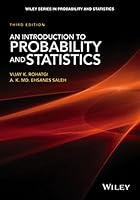# An Introduction to Probability and Statistics, 3rd Edition## Book Description

A well-balanced introduction to probability theory and

Featuring updated material, An Introduction to Probability and Statistics, Third Edition remains a solid overview to probability theory and mathematical statistics. Divided intothree parts, the Third Edition begins by presenting the and foundationsof probability. The second part addresses statistical inference, and the remainingchapters focus on special topics.

An Introduction to Probability and Statistics, Third Edition includes:

• A new section on regression to include multiple regression, logistic regression, and Poisson regression
• A reorganized chapter on large sample theory to emphasize the growing role of asymptotic statistics
• Additional topical coverage on bootstrapping, estimation procedures, and resampling
• Discussions on invariance, ancillary statistics, conjugate prior distributions, and invariant confidence intervals
• Over 550 problems and answers to most problems, as well as 350 worked out examples and 200 remarks
• Numerous figures to further illustrate examples and proofs throughout

An Introduction to Probability and Statistics, Third Edition is an ideal reference and resource for scientists and engineers in the fields of statistics, mathematics, physics, industrial , and engineering. The book is also an excellent text for upper-undergraduate and graduate-level students majoring in probability and statistics.

Chapter 1. Probability
Chapter 2. Random Variables and Their Probability Distributions
Chapter 3. Moments and Generating Functions
Chapter 4. Multiple Random Variables
Chapter 5. Some Special Distributions
Chapter 6. Sample Statistics and their Distributions
Chapter 7. Basic Asymptotics: Large Sample Theory
Chapter 8. Parametric Point Estimation
Chapter 9. Neyman-Pearson Theory of of Hypotheses
Chapter 10. Some Further Results on Hypotheses Testing
Chapter 11. Confidence Estimation
Chapter 12. General Linear Hypothesis
Chapter 13. Nonparametric Statistical Inference

## Book Details

• Title: An Introduction to Probability and Statistics, 3rd Edition
• Author: ,
• Length: 728 pages
• Edition: 3
• Language: English
• Publisher:
• Publication Date: 2015-09-08
• ISBN-10: 111879964X
• ISBN-13: 9781118799642